```<svg width="200px" height="200px" viewBox="0 0 200 200">
<!-- guide lines -->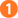<g style="stroke: gray; stroke-dasharray: 4 4;">
<line x1="0" y1="0" x2="200" y2="0"/>
<line x1="20" y1="0" x2="20" y2="90"/>
</g>

<g transform="translate(20, 0)">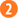<g transform="skewXskewY()">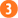<polyline points="50 0, 0 0, 0 50"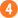style="fill: none; stroke: black; stroke-width: 2;"/>
<text x="0" y="60">skewX</text>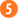</g>
</g>
</svg>```These dashed lines are drawn in the default coordinate system, before any transformation has occurred.This will move the entire skewed “package” to the desired location.Skew the coordinates by the given number of degrees. This transformation doesn’t change the origin, which will still be at (0, 0) in the new coordinate system.To make things easier, draw the object at the origin.Text will be covered in more detail in another chapter.

Zoom